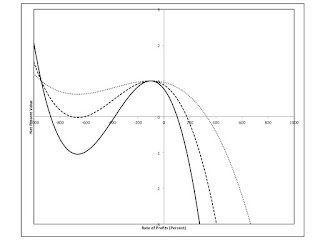## Tuesday, December 06, 2016

### Bifurcations In Multiple Interest Rate AnalysisFigure 1: Three Trinomials
1.0 Introduction

Typically, in calculating the Internal Rate of Return (IRR), a polynomial function arises. The IRR is the smallest, non-negative rate of profits, as calculated from a root of this function. The other roots are almost always ignored as having no economic meaning.

Michael Osborne, as I understand it, is pursuing a research project of investigating the use of all the roots of such polynomial functions that arise in financial analysis. A polynomial of degree n has n roots in the complex plane. I have noticed that the roots, other than the IRR, for examples that might arise in practice, can vary in whether they are real, repeating, or complex.

Bifurcation analysis, as developed for the study of dynamic systems might therefore have an application in multiple interest rate analysis. (This post is not about a dynamic system. I do not know how many of these results are about the theory of equations, independently of dynamical systems.) On the other hand, Osborne typically presents his analyses in terms of complex numbers. So I am not sure that he need care about these details.

2.0 An Example

Table 1 specifies the technology to be analyzed in this post. This technology produces an output of corn at the end of one specified year. The production of corn requires inputs of flows of labor in each of the three preceding years (and no other inputs). The labor inputs, per unit corn output, are listed in the table.

 YearBeforeOutput Labor Hiredfor Each Technique 1 L1 = 0.18 Person-Years 2 L2 = 4.468 Person-Years 3 L3 = 0.527438298 Person-Years

Let a unit of corn be the numeraire. Suppose firms face a wage of w and a rate of profits, r, to be used for time discounting. Wages are assumed to be advanced. That is, workers are paid at the start of the year for each year in which they supply flows of labor. Accumulate all costs to the end of the year in which the harvest occurs. Then the Net Present Value for this technology is:

NPV(r) = 1 - w[L1(1 + r) + L2(1 + r)2 + L3(1 + r)3]

The NPV is a third-degree polynomial. The wage can be considered a parameter. Figure 1, above, graphs this polynomial for three specific values of this parameters. In decreasing order, wages are 11/250, 11/500, and 2/250 bushels per person-years for these graphs.

Given the wage, the IRR is the intersection of the appropriate polynomial with the positive real axis in Figure 1. These IRRs are approximately 101.1%, 175.5%, and 329.5%, respectively. Suppose the economy were competitive, in the sense that capitalists can freely invest and disinvest in any industry. No barriers to entry exist. Then, if this technology is actually in use in producing corn and the wage were the independent variable, the rate of profits would tend to the IRR found for the wage. Profits and losses other than those earned at this rate of profits would be competed away.

The above graph suggests that, perhaps, the NPV for all wages intersects in two points, one of which is a local maximum. I do not know if this is so. Nor have I thought about why this might be. I guess it is fairly obvious that the local maximum is always at the same rate of profits. The wage drops out of the equation formed by setting the derivative of the NPV, with respect to the rate of profits, to zero.

I want to focus on the number of crossings of the real axis in the above graph. Figure 2 shows all roots of the polynomial equation defining the NPV. For a maximum wage, the IRR is zero, and it is greater to the right, along the real axis, for a smaller wage. The corresponding real roots, for the maximum wage, are the greatest and least negative rate of profits along the two loci shown in the left half of Figure 2. For smaller wages, these two real roots lie closer together, until around the middle wage used in constructing Figure 1, only one negative, repeated root exists. For any lower wage, the two roots that are not the IRR are complex conjugates. When the wage approaches zero, the workers live on air and all three roots go to (positive or negative) infinity.Figure 2: Multiple Rates of Profit for The Technique

This post has presented an example for thinking about multiple interest rate analysis. It is mainly a matter of raising questions. I do not know how the mathematics for investigating these questions impacts practical applications of multiple interest rate analysis.Anonymous said...

Are you using a physical conception of capital in your example? Leland Yeager writes,

"The trouble lies ... in searching for a purely physical conception of "capital" (or whatever it is whose yield or price is the rate of interest). The paradoxes dissolve when we recognize that the amount of that factor required in a physically specified production process does indeed depend on its own price"

Also, have you read the famous Keynes-Strachey correspondence? It's not supposed to be published until 2046, but it's been posted online. You'll learn a lot about Maynard Keynes.

sirroyharrod.blogspot.com

Robert Vienneau said...

Yeager is unreliable. Capital-theoretic issues with the neoclassical theory of value and distribution, including the Austrian variant do not disappear if one uses price-based measures of capital. And such measures cannot fill the role they were intended for in neoclassical theory.

Anyways, I use both physical specifications of stocks and flows in production and price-based measures of capital, as needed.

Thanks for the point to correspondence. I've read, quite a while ago, Keynes correspondence with Lydia. I imagine Keynes and Strachey would be quite explicit about the physical attributes of Duncan Grant and others.